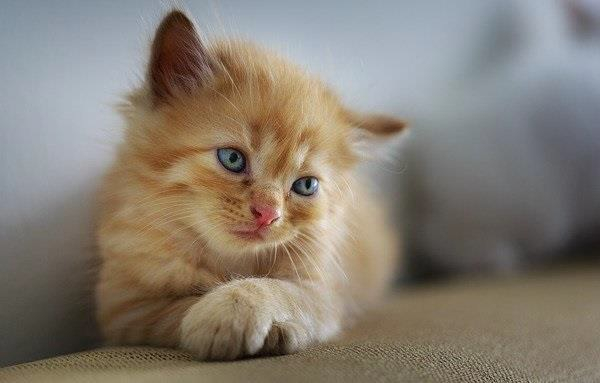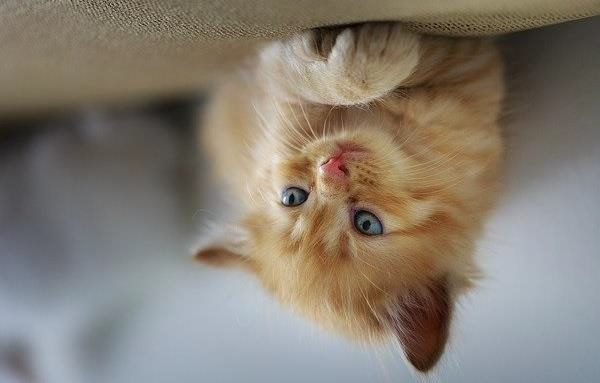# How to flip an image using Java OpenCV library?

The flip() method of the Core class of OpenCV flips an image along the x/y axis. This method accepts −

• A source matrix congaing the data of the original image.

• An empty destination matrix to hold the data of the resultant image.

• A flip code to specify the direction of the image (0 –x axis, +ve – y axis, – ve both the axes).

To flip an image −

• Read the contents of the image file to a matrix using the imread() method.

• Create an empty matric to hold the result.

• Invoke the flip() method by passing the above-created matrices.

• Create an image using the imwrite() method, bypassing the destination matrix as a parameter.

## Example

import org.opencv.core.Core;
import org.opencv.core.Mat;
import org.opencv.imgcodecs.Imgcodecs;
public class ChangingOrientation {
public static void main(String args[]) {
//Reading the Image from the file and storing it in to a Matrix object
String file ="D:\Images\cat.jpg";
//Creating an empty matrix to store the result
Mat dst = new Mat();
//Changing the orientation of an image
Core.flip(src, dst, -1);
//Writing the image
Imgcodecs.imwrite("D:\Images\flipping.jpg", dst);
System.out.println("Image Processed");
}
}

## Input## Output